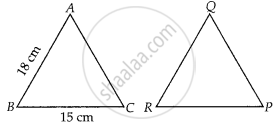# If ∆ABC ~ ∆QRP, ar(ABC)ar(PQR)=94, AB = 18 cm and BC = 15 cm, then PR is equal to ______ - Mathematics

MCQ
Fill in the Blanks

If ∆ABC ~ ∆QRP, (ar(ABC))/(ar(PQR)) = 9/4, AB = 18 cm and BC = 15 cm, then PR is equal to ______.

#### Options

• 10 cm

• 12 cm

• 20/3 cm

• 8 cm

#### Solution

If ∆ABC ~ ∆QRP, (ar(ABC))/(ar(PQR)) = 9/4, AB = 18 cm and BC = 15 cm, then PR is equal to 10 cm.

Explanation:

Given, ∆ABC ~ ∆QRP, AB = 18 cm and BC = 15 cmWe know that, the ratio of the area of two similar triangles is equal to the ratio of square of their corresponding sides.

∴ (ar(∆ABC))/(ar(∆QRP)) = (BC)^2/(RP)^2

But, (ar(∆ABC))/(ar(∆PQR)) = 9/4  .....[Given]

⇒ (15)^2/(RP)^2 = 9/4  .....[∵ BC = 15 cm, given]

⇒ (RP)^2 = (225 xx 4)/9 = 100

∴ RP = 10 cm

Concept: Similarity of Triangles
Is there an error in this question or solution?

#### APPEARS IN

NCERT Mathematics Exemplar Class 10
Chapter 6 Triangles
Exercise 6.1 | Q 11 | Page 62
Share